# Finding Reduced Basis for LatticesPage 1

#### WATCH ALL SLIDES

Slide 1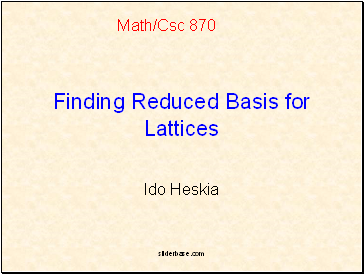Finding Reduced Basis for Lattices

Ido Heskia

Math/Csc 870

Slide 2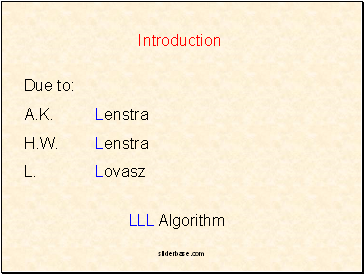Due to:

A.K. Lenstra

H.W. Lenstra

L. Lovasz

LLL Algorith

Introduction

Slide 3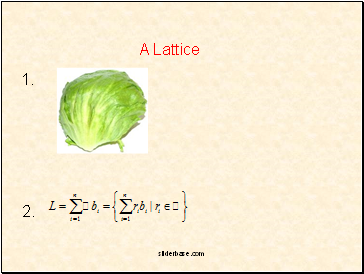## A Lattice

1.

2.

Slide 4Let n be a positive integer. A subset L of the n-dimensional real vector space is

called a lattice if there exists a basis b1,b2,…,bn of such that

The bi’s span L.

n is the rank of L.

We will consider only

Slide 5Constructing lattices:

Slide 6Determinant of L:

The bi’s are written as column

vectors. Apparently, this positive

real number doesn’t depend on the

choice of the basis.

Slide 7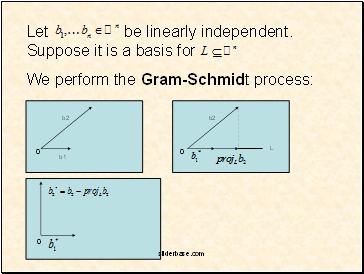Let be linearly independent. Suppose it is a basis for

We perform the Gram-Schmidt process:

b1

b2

b2

0

0

L

0

Slide 8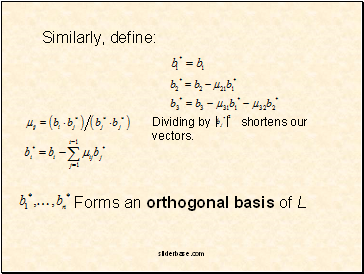Similarly, define:

Forms an orthogonal basis of L

Dividing by shortens our vectors.

Slide 9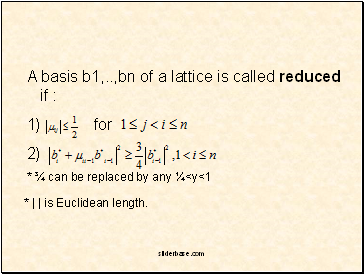A basis b1, ,bn of a lattice is called reduced if :

for

* ¾ can be replaced by any ¼<y<1

* | | is Euclidean length.

Slide 10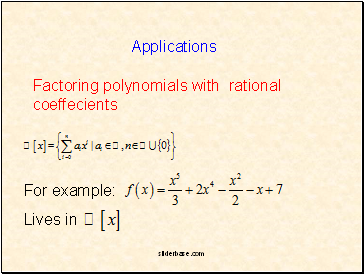## Applications

Factoring polynomials with rational coeffecients

For example:

Lives in

Slide 11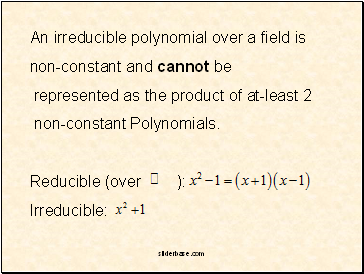An irreducible polynomial over a field is

non-constant and cannot be

represented as the product of at-least 2

non-constant Polynomials.

Reducible (over ):

Irreducible:

Slide 12How to find, for a given non-zero

polynomial in its decomposition into

Irreducibles?

Factor primitive polynomials

(gcd of all coeffecients of f is 1)

Into irreducible factors in

Use LLL

Slide 13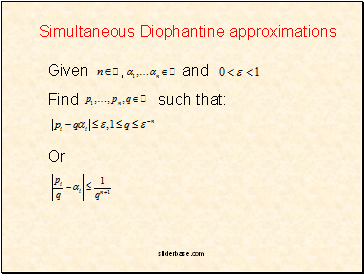## Simultaneous Diophantine approximations

Given , and

Find such that:

Or

Slide 14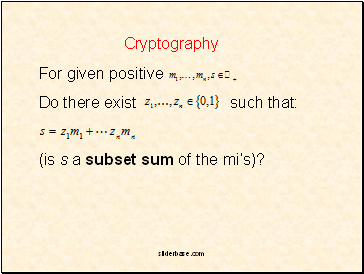Go to page:
1  2